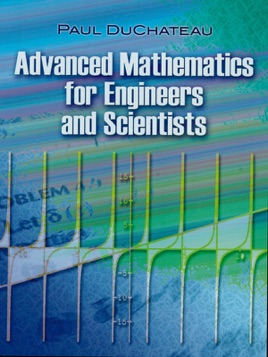• USD 19.99

## Descripción de editorial

This book can be used as either a primary text or a supplemental reference for courses in applied mathematics. Its core chapters are devoted to linear algebra, calculus, and ordinary differential equations. Additional topics include partial differential equations and approximation methods.
Each chapter features an ample selection of solved problems. These problems were chosen to illustrate not only how to solve various algebraic and differential equations but also how to interpret the solutions in order to gain insight into the behavior of the system modeled by the equation. In addition to the worked-out problems, numerous examples and exercises appear throughout the text.